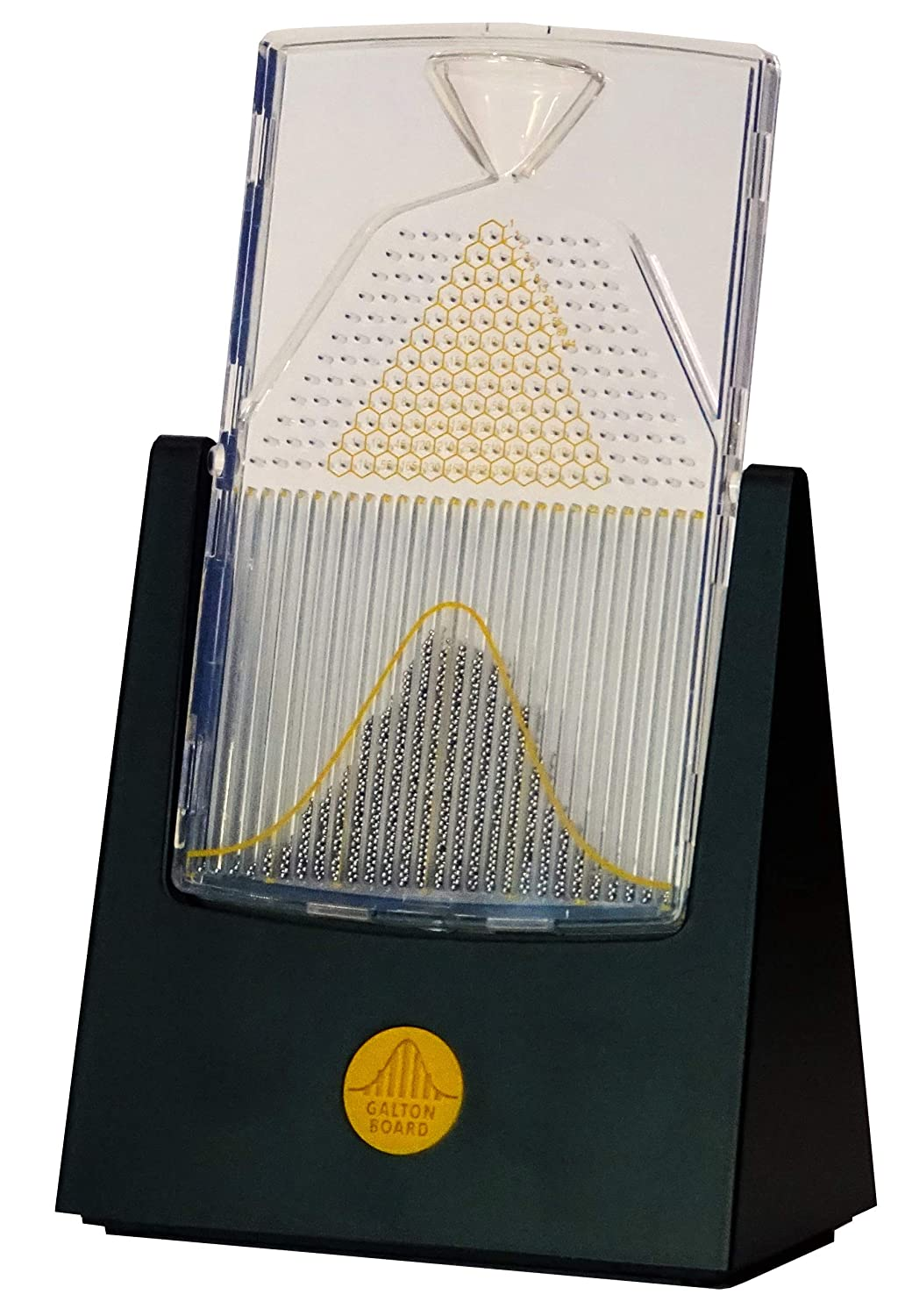# Galton Board - Math In Motion

Regular price \$89.70

: In stock

:

\$89.70

### Have a Question?

Four Pines Publishing is making new Galton Boards now and there will be an equal distance between the std dev lines. It will also have a smaller single brass bead so it will not occasionally get lodged in bin 12. New boards should be available around early March. The Galton Board is math in motion, demonstrating centuries-old mathematical concepts in an innovative desktop device.  It also has a superimposed Pascal’s Triangle, which is a triangle of numbers that follows the rule of adding the two numbers above to get the number below. The number at each peg represents the number of different paths a bead could travel from the top peg to that peg.

The Fibonacci numbers can also be found as the sums of specific diagonals in the triangle. When rotated on its axis, the 3,000 beads cascade through rows of symmetrically placed pegs in the desktop-sized Galton Board. When the device is level, each bead bounces off the pegs with equal probability of moving to the left or right. As the beads settle into the bins at the bottom of the board, they accumulate in approximately a bell curve. Printed on the board are the bell curve, the average, and standard deviation lines. The bell curve, also known as the Gaussian distribution, is important in statistics and probability theory. It is used in the natural and social sciences to represent random variables, like the beads in the Galton Board. The Galton Board is reminiscent of Charles and Ray Eames’ groundbreaking 11-foot-tall “Probability Machine,” featured at the 1961 Mathematica exhibit. An even larger Eames probability machine was showcased at IBM’s Pavilion for the 1964 World’s Fair in New York. Both the Galton Board and the superimposed Pascal’s Triangle incorporate many related mathematical, statistical, and probability concepts. The Galton Board is 100% satisfaction guaranteed!

In the Galton Board you may see: the Gaussian curve of the normal distribution, or bell-shaped curve; the central limit theorem (the de Moivre-Laplace theorem); the binomial distribution (Bernoulli distribution); regression to the mean; probabilities such as coin flipping and stock market returns; the law of frequency of errors; and what Sir Francis Galton referred to as the “law of unreason.”